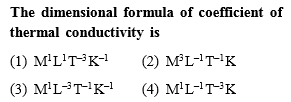Concept Videos :-

#1 | Basic Concepts & Examples
#2 | Dimensional Analysis : Remaining

Concept Questions :-

Dimensions

(1)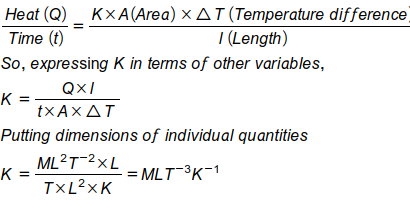Where K is thermal conductivity

Difficulty Level:

• 45%
• 26%
• 23%
• 7%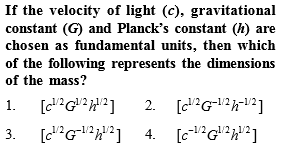Concept Videos :-

#1 | Basic Concepts & Examples
#2 | Dimensional Analysis : Remaining

Concept Questions :-

Dimensions

Difficulty Level:

• 21%
• 30%
• 42%
• 8%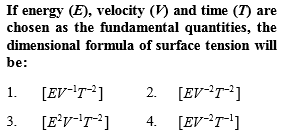Concept Videos :-

#1 | Basic Concepts & Examples
#2 | Dimensional Analysis : Remaining

Concept Questions :-

Dimensions

Difficulty Level:

• 16%
• 58%
• 18%
• 11%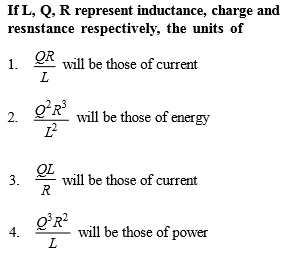[Only for Dropper batch]

Concept Videos :-

#1 | Basic Concepts & Examples
#2 | Dimensional Analysis : Remaining

Concept Questions :-

Dimensions

Also,

so,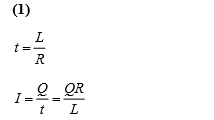Difficulty Level:

• 44%
• 24%
• 26%
• 9%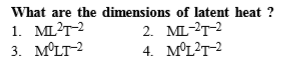Concept Videos :-

#1 | Basic Concepts & Examples
#2 | Dimensional Analysis : Remaining

Concept Questions :-

Dimensions

(4)

$Q=mL\phantom{\rule{0ex}{0ex}}L=\frac{Q}{m}=\frac{\left[M{L}^{2}{T}^{-2}\right]}{\left[M\right]}=\left[{M}^{0}{L}^{2}{T}^{-2}\right]$

Difficulty Level:

• 29%
• 19%
• 18%
• 36%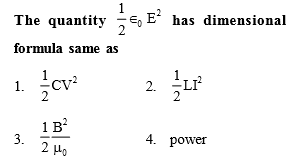[Only for dropper batch]

Concept Videos :-

#1 | Basic Concepts & Examples
#2 | Dimensional Analysis : Remaining

Concept Questions :-

Dimensions

(3)

Difficulty Level:

• 27%
• 18%
• 40%
• 18%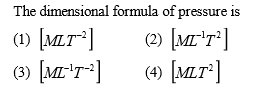Concept Videos :-

#1 | Basic Concepts & Examples
#2 | Dimensional Analysis : Remaining

Concept Questions :-

Dimensions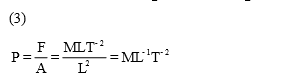Difficulty Level:

• 12%
• 21%
• 66%
• 4%

Light year is a unit of

(1) Time

(2) Mass

(3) Distance

(4) Energy

Concept Videos :-

#1 | Basic Concepts & Examples
#2 | Dimensional Analysis : Remaining

Concept Questions :-

Dimensions

(3) Light year is a distance which light travels in one year.

Difficulty Level:

• 24%
• 6%
• 64%
• 8%

The magnitude of any physical quantity

(1) Depends on the method of measurement

(2) Does not depend on the method of measurement

(3) Is more in SI system than in CGS system

(4) Directly proportional to the fundamental units of mass, length and time

Concept Videos :-

#1 | Basic Concepts & Examples
#2 | Dimensional Analysis : Remaining

Concept Questions :-

Dimensions

(2) Because magnitude is absolute.

Difficulty Level:

• 26%
• 27%
• 13%
• 36%

Which of the following is not equal to watt

(1) Joule/second

(2) Ampere $×$ volt

(3) (Ampere)2 $×$ ohm

(4) Ampere/volt

Concept Videos :-

#1 | Basic Concepts & Examples
#2 | Dimensional Analysis : Remaining

Concept Questions :-

Dimensions

(4)

Difficulty Level:

• 22%
• 16%
• 29%
• 35%